## The ABC's of Physics

### The ABC’s of Physics

Before we dive into forces, gravitational or otherwise, we should understand the underlying concepts of motion in a framework of space and time. We need a foundation on which to build our knowledge of forces, or physics. Same difference. What is physics but the study of forces and the motion they cause?

### Space & Time

If we’ll study how things move, then there are a few terms we need to become familiar with, or more familiar with. First up: space.

We’re all familiar with the space around us. It’s everywhere, all encompassing, and we can fit everything in it. Space could be something simpler than that though. Something as simple as a straight line, like a wire. The complexity of space depends on the number of dimensions it has.

A wire is one dimensional, so beads stringed by it would be bounded by the wire’s one dimension. Since the wire only provides one dimension for the beads to move in, the beads could only move up or down if the wire stretches up and down; they could move side to side, but only if the stretches from side to side, too; the beads could not move up and down and side to side at the same time. A dimension is the range of possible motion. If it’s only in one line or on one axis, then it’s one dimensional.

Space can have more than one dimension, such as a chessboard with pieces that can move in the various directions along the surface of the board, making two dimensions, usually labeled x and y. Space can also be like the 3-dimensional space that we know and love and live in, with objects having length, width, and height.

A bird flying around in 3D space flies up and down and to one side or the other, back and forth, then it puts its right foot out and shakes it all around. However, it gets where it’s going, our bird travels some distance. To get from one point on the wire to another, a bead slides some amount, or distance. To get from one branch to another, a bird flies and hops another certain distance.

The standard international unit (SI unit) of distance is the meter (m). Other distance units are feet, inches, centimeters, miles, kilometers, etc. These other types of distance units may be converted to the SI unit of meters, such as 2.54 centimeters in an inch, and 100 centimeters in a meter.

Here’s a question. Ignoring any possible changes in height above the ground, if a bird flies within two dimensions 5 m West, 5 m South, 5 m East, and 5 m North, what total distance d does it fly?

Go ahead, think about it. Lalala…That’s right. All we have do is add up each individual distance to get the total distance d, so d = 5 + 5 + 5 + 5 = 20 m.

If we think of the bird’s position a different way, we realize that it ends right back where it started. The bird has no displacement. Displacement measures the distance between the final and initial positions.

The only thing we’re missing now is the concept of direction. Funny, that’s what our career counselor said, too—before we got into Shmooping, that is. Let’s say the wired bead slid 5 centimeters from where it started, but we don’t know which way it went.

In terms of math, we need to add a sign convention to our concept of distance. If we say the bead started at position zero, then it either went +5 centimeters or -5 centimeters from that zero position. Of course, it’s entirely up to us to decide which way will be positive and which way will be negative. Previous math classes predilect us to choose left or down for negative and right or up for positive, but it’s truly inconsequential as long as we’re consistent.

Tweetie, on the other hand, travels in two dimensions. He went side to side and he went up and down too. We’ve seen this in math class before as Cartesian Coordinates, where each of the two dimensions of measurements are plotted on the y- and x-axes of a graph.

Now we understand the dimensions of space that objects move in, whether it’s a string, a chess board, or a room bounded by spatial dimensions of distance (assuming the room is clean enough to move around in…ours not so much). We, along with everything else, however, also move in time. We can say that Tweetie moved from point A to point B on his first leg, 5 m away to the West. We can also say that he spent exactly 1 second flying each of his four legs because Tweetie is crazy fast like that.

Time is very similar to space, except, of course, that we can’t see it, making it quite a bit harder to visualize. Think of time as an invisible line on which we have no choice but to move forwards. Time has only one dimension to travel along; there’s no concept of horizontal or vertical time, at least that we can tell. We move from 5:10:00 p.m. to 5:10:01 p.m. along the dimension of time by staying put. Who said we’d never go anywhere?

We may also plot time as one of the coordinates on a Cartesian plot. Time is always plotted on the x-axis. That’s because time is the most independentiest of all dimensions, that is to say, all motion depends on time yet time depends on nothing.

Here’s Tweetie’s voyage graphed numerically as displacement over time, in units of seconds, which also happens to be the SI unit for time. Recall that Tweetie spent only 1 second on each leg of his journey.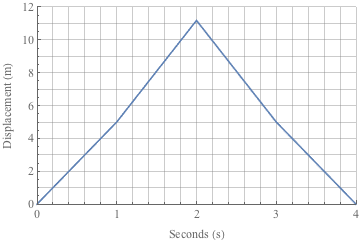Note that we can’t graph three dimensions simultaneously on a Cartesian grid. That means we can graph displacement versus time because that’s two dimensions, but we can’t graph position versus time because that’s three dimensions. The displacement, again, is the distance from whatever point Tweetie’s at any given time from the origin, where he started.

Notice that time can be negative? If a time precedes the chosen “time zero”, then it’s required to be negative. Now, if only we could move in the negative time direction, we’d be time traveling, and that would be more awesome than using the force.

Working together, the identity of both space and time together set up events, stories, and accidents. If friends plan to meet at the library on Wednesday, they may all arrive at the spatial position of the library but not see each other because of the differences in time of arrival. Together, we call this spacetime. That’s why we lump the discussion of time together with space.

### Speed

Without naming it, we have already touched on the next concept on the list, speed, which is distance over time or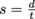. Tweetie’s speed is 5 meters in 1 second, or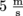, the SI units for speed.

For alternative units, all we have to do is think of a car’s speedometer: it outputs speed in miles per hour. Miles is a unit of distance and hour is a unit of time. For instances when the beginning distance or time isn’t zero, we may find the speed by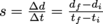, where “f” stands for final and “i” for initial. Not “initial” as in a monogram, but “beginning.”

That graph we saw earlier of Tweetie’s displacement over time doesn’t help us calculate speed because magnitude of displacement isn’t the total distance traveled but the distance from the beginning position. Speaking of Tweetie, how fast does he fly as he takes 1 second to fly each of his four 5 m legs?

Tweetie travels 5 meters in 1 second, or 20 meters in 4 seconds. Either way, we divide the distance by the change in time to get a speed of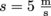. We could convert from meters per second to miles per hour if we chose, and why not? The conversion would put the speed in familiar terms.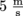is 11.2 mph, faster than the fastest the average human can run.

Note that Tweetie’s speed ofprobably isn’t exactly the same on the corners of his path. What we’ve actually measured is the average speed, not a precise speed for each moment during all 4 seconds of flight. Small difference, but in that difference lies the difference between studying algebra and studying calculus.

Let’s change it up. If Tweetie stopped for 3 seconds to smooth out his plumage on a branch in between two of the legs of his journey (why, oh why, are they called legs?) what’s his average speed for the entire voyage with his beauty rest?

We want the total distance over the total time for an average speed. Total distance stays the same, since Tweetie doesn’t travel anywhere when he grooms himself. His trip, however, now lasts a total of (4 + 3) = 7 seconds. Therefore, the average speed is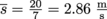. That line over “s” denotes that it’s an average speed, also possibly described by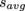.

The next time someone asks for the average speed of anything, all that matters is the total distance traveled and the total time it took, regardless of how many stops and changes in direction happened along the way, from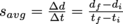.

However, when police officers ask their typical, “do you know how fast you were going?” question, they don’t mean average speed. Let’s just agree to not bring it up. They wouldn’t appreciate a scientific discussion right then, and we wouldn’t want to make any more trouble for ourselves.

Like police officers, physicists don’t exclusively use the concept of average speed. Objects change their speed and direction all the time. To deal with this, we can introduce the concept of instantaneous speed, which is the exact speed that an object is traveling at any given point in time.

Tweetie’s case is relatively simple, since he stopped only once between two legs to get all prettied up. At that point his speed was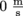. During most of each leg, though, he was traveling at a speed of 5 m/s. Tweetie can’t go from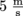to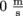instantaneously, unless he’s some sort of mutant. We’ll address changes in speed soon, but not yet.

### Scalars vs Vectors and Speed vs Velocity

Before we talk more about changes in speed, we need to bring direction into the idea of speed.

Speed is great if we just want to know how fast Tweetie flies, but what if we want to know his direction? Speed alone is not good enough. To add direction to speed, we leap from a scalar quantity, a number, to a vector quantity: a quantity with direction. In the case of speed, we call speed and direction together velocity.

A vector is symbolized as an arrow of a certain length. The length corresponds to the quantity. The direction of the arrow corresponds to…the direction. That means that the length, a.k.a. the scalar, can represent speed, distance, or something else. The direction of the arrow turns that scalar into velocity, displacement, or something else, respectively. Yes, displacement has a direction, so it’s a vector too. Minds: blown.

Vectors exist in one, two, or three dimensions of space. In one dimension, there are only two possible directions for an arrow to point. In two dimensions, the arrow might point any direction a compass could—the direction is generally measured by angles rather than North or West or whatever. In three dimensions, vectors can point anywhere from the center of a sphere outward.

We can graph the individual displacements of Tweetie’s trip as vectors in two dimensions, for example, for a net displacement of 0, which doesn’t have an arrow because zero is, well, zero. Again, the length of the arrow shows the length of the distance while the direction on the arrow points the direction.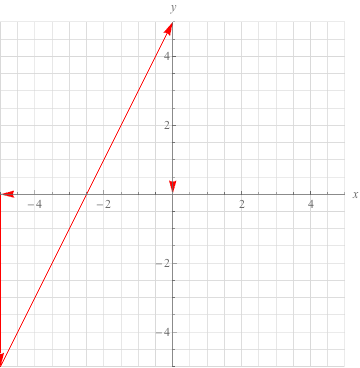We don’t have to worry about the three-dimensional vectors right now. For the purposes of this study guide, velocity is a two-dimensional vector, as is displacement and acceleration. They may change in x- or y-directions or some combination of the two. Many times we’ll address the x- and y-directions separately, in one dimension, before combining them to make them 2D from the Pythagorean Theorem.

We can’t say there was no warning.

We denote vectors by bolding the their scalar counterparts or by writing an arrow over the top of the scalar, such as d (the scalar) versus d or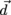. For velocity, we traditionally don’t use s, but v or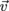. Get it? Got it? Good.

The final thing to know is denoting magnitude of a vector quantity such as |v|or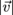in the case of velocity. For velocity and speed, s = |v|. Usually we just call speed v, the unbolded, un-arrowed version. Forget s, because you’ll only see v from here on out.

Knowing, as we do now, that displacement is actually a vector, we need to change up the graph of Tweetie’s displacement over time. Before, it was this: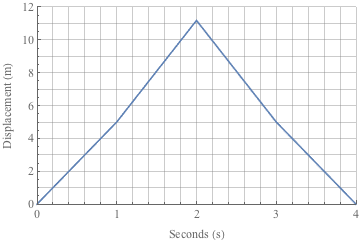Now that we know about vectors, we identify this as the graph of |d|, the magnitude of the displacement. If we graph d (the same as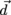) as a function of time, we have a problem: both x- and y-directions have displacement, but we can only graph one of them as a function of time at a time. That’s confusing.

Instead of trying to do that confusing thing, we’ll separate the displacement into two: the x-displacement, and the y-displacement,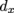and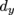, and graph those. Yup, we’re treating a two-dimensional entity (displacement) as two one-dimensional parts.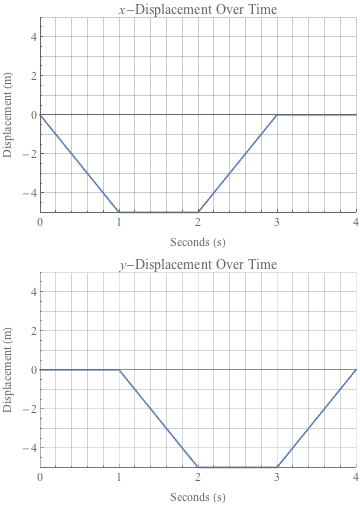Ah, that makes sense now. Now, let’s turn our attention to velocity v, the vector version of speed v. It’s too bad “speed” doesn’t start with a “v”.

When Tweetie flew from East to West, his velocity was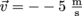in the x-direction, but his speed was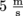. When he flew from West to East, his velocity was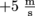and his speed was also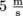, in the x-direction, otherwise known as “x-hat”,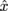. Concisely, we’d write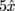. The same is true for North and South in the y-direction,, said “y-hat”.

Instead of describing vectors, we usually draw them, with the speed as the number over the arrow, like this for the Eastern leg of Tweetie’s flight.### Acceleration

Last but certainly not least, we’ll study changes in speed or velocity. When a change in speed (or velocity) occurs in some amount of time, it’s called acceleration, or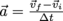. For example, after Tweetie finished primping, his speed gradually accelerated from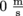to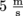.

Considering the legs of Tweetie’s journey only lasted a second, we may assume that in his case, the change in speed happened in a fraction of a second, say 0.1 seconds. We may now calculate the acceleration as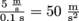.

Unit alert. Unit alert. Note the units on that acceleration: dividing a rate by time again makes “seconds squared” in the unit denominator. That’s important because without squared time in the unit denominator, there’s no acceleration.

Change in speed doesn’t have to be an increase—acceleration decreases too, and we call that deceleration. When Tweetie slowed down, he decelerated.

After his now notorious and repetitive journey, Tweetie notices the crowd of Shmoopers watching his every movement and taking notes. He decides to take his sweet time slowing down before embarking on his grooming mission this time around. As he flaunts himself, his velocity decreases from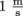to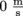in 10 seconds while traveling in the x-direction. What’s his average acceleration?

All we have to compute is by how much Tweetie’s velocity changed in the given amount of time, so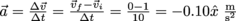. Don’t forget that x-hat.

Is it true that if an object has a negative acceleration, it is decelerating with its speed decreasing)?

The answer is no. If west is negative, for example, and Tweetie were to go from flying at a velocity of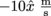to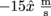in one second, then we’d end up with an acceleration of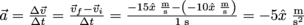. That’s despite the fact that Tweetie’s speed, found from velocity by taking the magnitude alone as |v|, is clearly increasing. Directions matter with vectors.

Conversely, is it true that if an object is decelerating, it must have a negative acceleration? The answer is also no. For example, if Tweetie were to go from flying at a velocity ofto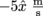, we’d end up with an acceleration of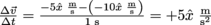. This, despite the fact that Tweetie clearly lost some wind up there.

Just remember we’re allowed to pick West as positive instead, so what really matters overall is whether something is moving faster or slower than it was just before, regardless of which way it’s heading. However, we have to be consistent. Both West and East can’t be positive in the same problem, though both West and East would be noted with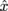, and North and South with positive or negative. Or we can use y for up and down, and x for some side to side not aligned with any mark on a compass. It doesn’t matter, as long as we’re consistent.

Here’s a graph of what acceleration is in comparison to a one-dimensional displacement and velocity.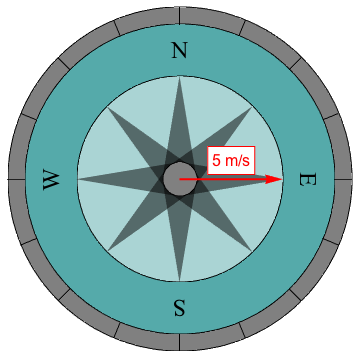Source

In the graph above, we see that when displacement is increasing steeply in the positive direction, velocity increases steadily, meaning a steady (horizontal) acceleration. A straight line of displacement means a flat line of velocity and zero acceleration. Slowing down decreases velocity with a negative acceleration. Standing still at one position means no velocity or acceleration whatsoever.

Moving in the negative direction with a decreasing position creates a negative velocity and acceleration. Lowering the displacement steadily produces a constant negative velocity but no acceleration because there’s no change in speed or direction. And last, but certainly not least, when the negative displacement slows, we get velocity increasing (by being less negative, approaching zero) and a positive acceleration.

Each of these relationships may be verified mathematically by assigning some numbers to the displacement graph. If we care to go to that effort.

### Common Mistakes

Review the whole scalar/vector business with every sunrise until the whole positive vs. negative acceleration thing makes sense. Also: notation, notation, notation. A vector such as velocity is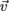or v. The magnitude of a vector such as velocity is |v|, which identical to the speed s in the case of velocity. The average speed (or average velocity, etc.) is either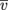or vavg, or in the case of vector form then vavg or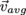.

Lastly, don’t forget the units, or the hats. They both matter a whole lot in physics. A velocity of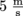in the positive x-direction would be written more concisely as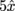.

### Brain Snack

When gathering evidence of the Copernicus’ heliocentric view of the solar system, Galileo also discovered that if moving at constant velocity, such as in a ship, its seated occupants may believe that they’re at rest, and therefore if Earth were moving at a constant velocity around the Sun, its inhabitants wouldn’t notice that motion either.